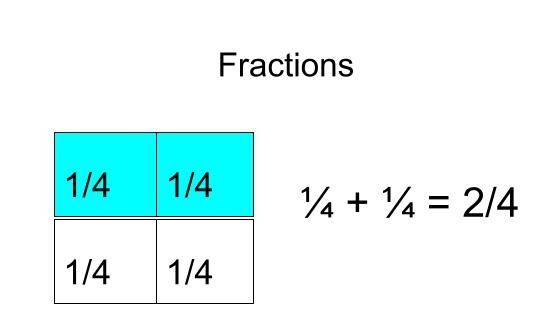# Fractions# SUMMARY

Face to Face lesson

In this lesson students will learn what a fraction is, how to identify a fraction, and the parts of a fraction; The numerator and denominator.  Students will be able to compare fractions with <, >, =.  Students will then learn how to add and subtract fractions with common denominators.

1 of 7